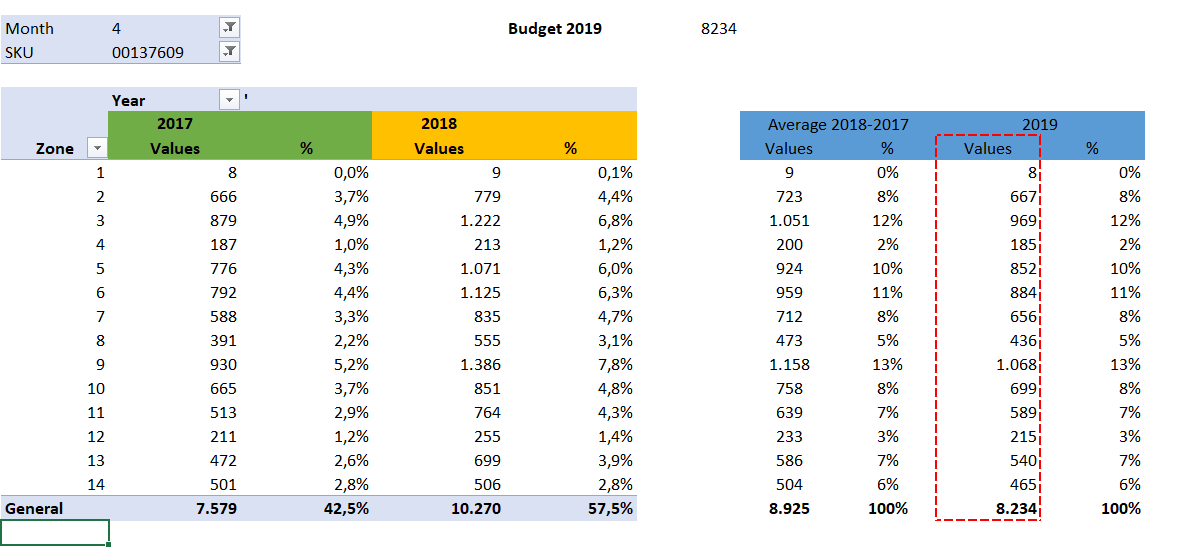# Help with data split

Member Posts: 5Contributor I
edited December 2018 in Help
Hi! I need help with data split.
I have a monthly budget for each product and I need to do a data-split for different geographical zones, according to historical company records.
For example, for a specific SKU there is a budget of 100 units a month. historically, the units are divided this way: Zone 1: 30%, zone 2: 20% and zone 3: 50%. So the budget for each Zone would be 30, 20 and 50 for zones 1, 2 and 3 respectively.

(that's just an example, I need to work with aprox 1500 SKU in 200 zones during 12 months)

Thank you

## Answers

• Member Posts: 5Contributor I
edited December 2018
Hi @lionelderkrikor

I have historical monthly sales data from 2017 and 2018 for each sku and sales zones.
Also I have the budget 2019 for each sku, but I don't have budget for each sales zones.
I need divide the 2019 budget for each zone according to the historical sales data.

I attach an example of excelThanks!

• Moderator, RapidMiner Certified Analyst, RapidMiner Certified Expert, Member Posts: 1,125Unicorn
If you simply want to compute these percentages in RapidMiner, you can do that first by generating the average or sum of the prior years by zone with Generate Attrbiutes, and then use the Aggregate operator, where you group by Zone and then calculate the sum(fractional) as your aggregation function on your created variable.
If you want to do something more complicated, like build a separate forecast model for each zone, then you could do that as well, but probably you would need to use Loop to go through the different values for zone and apply a separate forecast process to each.

Brian T.
Lindon Ventures
Data Science Consulting from Certified RapidMiner Experts
• Member Posts: 5Contributor I
Hi @Telcontar120

How could I do this?
I have historical units and total budget units as input for each SKU

Thanks!!
• Moderator, RapidMiner Certified Analyst, RapidMiner Certified Expert, Member Posts: 1,125Unicorn
Have you created a process yet in RapidMiner?  Did you take a look at the Aggregate operator sample process and compare it?  You should post your process here with a a data sample and be more specific about the problem you are encountering.  I provided a general description of how you would do this in RapidMiner already, but without a process to look at, I am not sure how I can be more helpful.
Brian T.
Lindon Ventures
Data Science Consulting from Certified RapidMiner Experts
• Member Posts: 5Contributor I
Hi @Telcontar120
I don't know which is the operator that I should use to make the data cross between the historical data and the budget data.
• Moderator, RapidMiner Certified Analyst, Member Posts: 716Unicorn
Hi @matias_sk2,

I have difficulties to understand what is your goal :
I know you provided a sample with your xls file but can you provide a more explicit explanation :
Can you provide an example of what you have, and an example of what you want to obtain (the relationship(s) between 2017/2018 and 2019)?

Regards,

Lionel
• Member Posts: 5Contributor I
Hi @lionelderkrikor

In the Excel attachment you can see the logic that you would use to calculate this. Please your help, in Excel this can't be done for the SKU number that I need to make the division (around 1500 SKU, in 2000 zones and for 12 months)

Thank you very much!
• Moderator, RapidMiner Certified Analyst, Member Posts: 716Unicorn
edited December 2018
Hi @matias_sk2 ,

Does this process answer to your need ?

```<?xml version="1.0" encoding="UTF-8"?><process version="9.1.000">
<context>
<input/>
<output/>
<macros/>
</context>
<operator activated="true" class="process" compatibility="9.1.000" expanded="true" name="Process">
<parameter key="logverbosity" value="init"/>
<parameter key="random_seed" value="2001"/>
<parameter key="send_mail" value="never"/>
<parameter key="notification_email" value=""/>
<parameter key="process_duration_for_mail" value="30"/>
<parameter key="encoding" value="SYSTEM"/>
<process expanded="true">
<operator activated="true" class="read_excel" compatibility="9.1.000" expanded="true" height="68" name="Read Excel" width="90" x="112" y="85">
<parameter key="excel_file" value="C:\Users\Lionel\Documents\Formations_DataScience\Rapidminer\Tests_Rapidminer\Budget_sku_zone\Budget_by_zone.xlsx"/>
<parameter key="sheet_selection" value="sheet number"/>
<parameter key="sheet_number" value="2"/>
<parameter key="imported_cell_range" value="O5"/>
<parameter key="encoding" value="SYSTEM"/>
<parameter key="first_row_as_names" value="true"/>
<list key="annotations"/>
<parameter key="date_format" value=""/>
<parameter key="time_zone" value="SYSTEM"/>
<parameter key="locale" value="English (United States)"/>
<parameter key="read_all_values_as_polynominal" value="false"/>
<list key="data_set_meta_data_information"/>
<parameter key="read_not_matching_values_as_missings" value="true"/>
<parameter key="datamanagement" value="double_array"/>
<parameter key="data_management" value="auto"/>
</operator>
<operator activated="true" class="aggregate" compatibility="9.1.000" expanded="true" height="82" name="Aggregate" width="90" x="246" y="85">
<parameter key="use_default_aggregation" value="false"/>
<parameter key="attribute_filter_type" value="all"/>
<parameter key="attribute" value=""/>
<parameter key="attributes" value=""/>
<parameter key="use_except_expression" value="false"/>
<parameter key="value_type" value="attribute_value"/>
<parameter key="use_value_type_exception" value="false"/>
<parameter key="except_value_type" value="time"/>
<parameter key="block_type" value="attribute_block"/>
<parameter key="use_block_type_exception" value="false"/>
<parameter key="except_block_type" value="value_matrix_row_start"/>
<parameter key="invert_selection" value="false"/>
<parameter key="include_special_attributes" value="false"/>
<parameter key="default_aggregation_function" value="average"/>
<list key="aggregation_attributes">
<parameter key="Units 2019" value="sum"/>
</list>
<parameter key="group_by_attributes" value="Zone"/>
<parameter key="count_all_combinations" value="false"/>
<parameter key="only_distinct" value="false"/>
<parameter key="ignore_missings" value="true"/>
</operator>
<connect from_op="Read Excel" from_port="output" to_op="Aggregate" to_port="example set input"/>
<connect from_op="Aggregate" from_port="example set output" to_port="result 1"/>
<portSpacing port="source_input 1" spacing="0"/>
<portSpacing port="sink_result 1" spacing="0"/>
<portSpacing port="sink_result 2" spacing="0"/>
</process>
</operator>
</process>
```

Hope it helps,

Regards,

Lionel

Sign In or Register to comment.